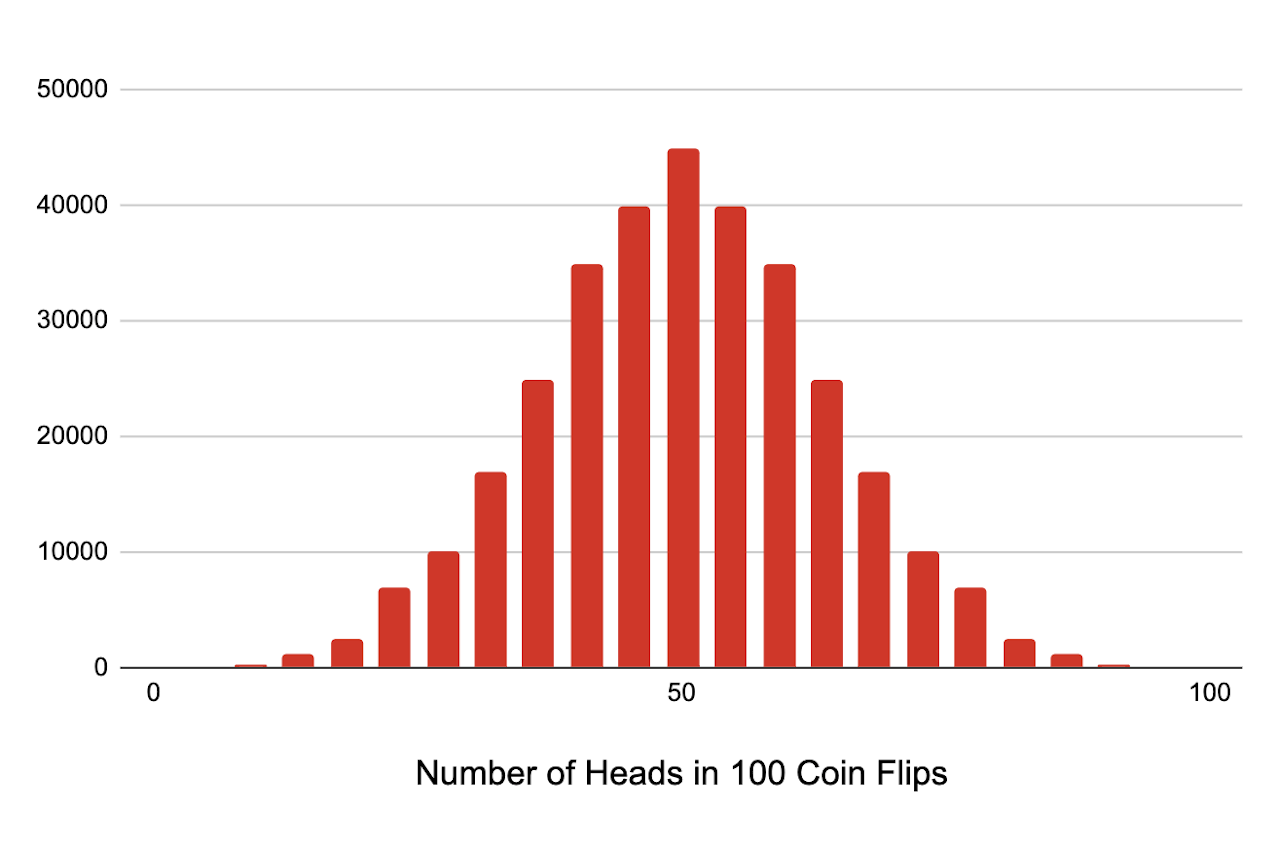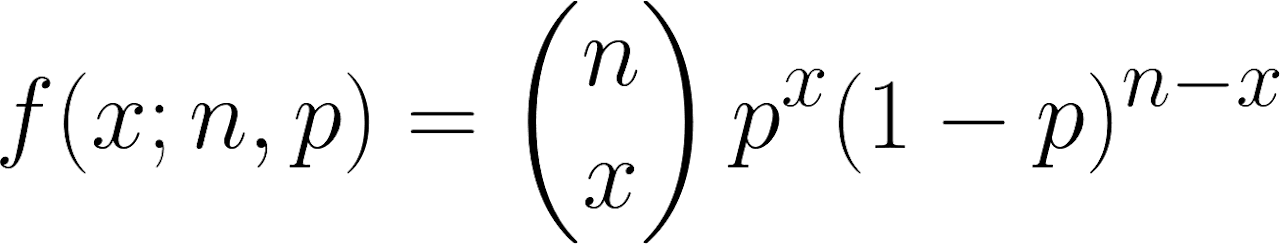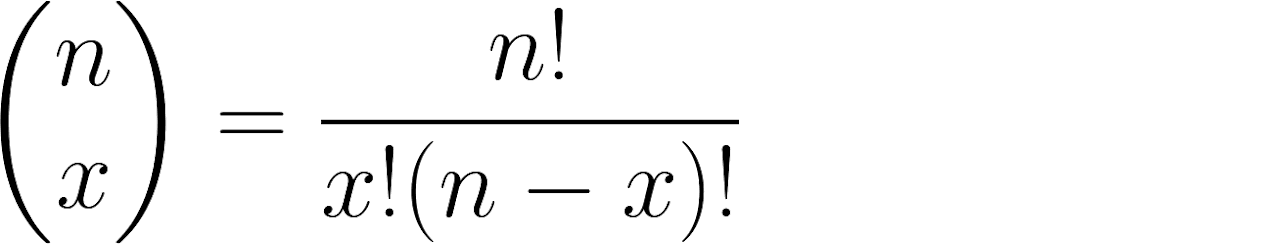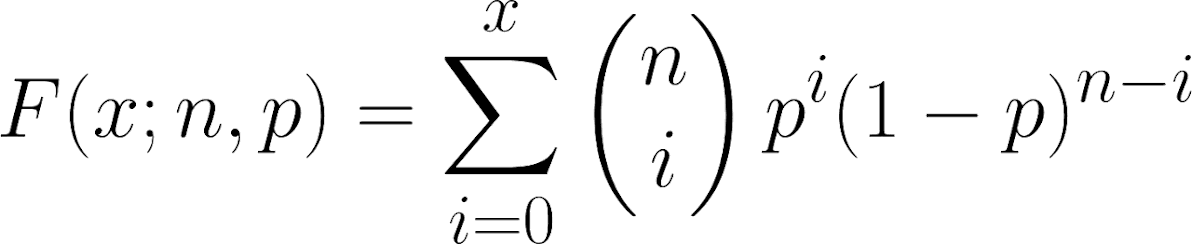# Binomial Distribution Calculator

Enter the probability of success for a single trial, the number of trials, and the number of successes to calculate the binomial and cumulative probabilities of getting successful events.

## Probabilities:

Learn how we calculated this below

## What is a Binomial Distribution?

In statistics, a binomial distribution is a probability distribution of the number of successes in a sequence of independent experiments. The result of each experiment must be boolean, which means the result must be a yes/no or success/fail.

Unlike a normal distribution, a binomial distribution is a probability distribution for a discrete variable, while a normal distribution is a distribution for a continuous variable. Binomial distributions can also be skewed, while a normal distribution is always symmetrical.

Each experiment in the sequence is referred to as a trial, or more specifically, a Bernoulli trial. The sequence is sometimes referred to as a Bernoulli process, named for mathematician Jacob Bernoulli.

When the results of x success in the sequence of n trials with a probability p of success in a single trial are plotted, they form a distribution curve.

To help better understand the binomial distribution, let’s use the example of recording coin flips. Let’s say we ran a sequence of 100 coin flips and measured the number of times it landed on heads. If we ran this sequence 250,000 times and plotted the number of heads in each sequence, we might see a distribution like this one.In this sequence, the number of trials n is 100, representing the 100 coin flips. The number of successes x is the number of times in the sequence that the coin landed on heads. The probability p is 0.5 since there is a 50% chance of landing on heads.

## How to Calculate a Binomial Probability

A binomial probability is a probability of getting exactly x successes in a sequence of n trials, where the probability p of success in each trial is the same. Each trial must be independent and mutually exclusive.

### Binomial Probability Formula

You can calculate the binomial probability using a formula:Thus, the probability of x successes in a sequence of n trials with the probability p of success in each trial is equal to the number of possible combinations of success times p to the power of x times 1 minus p to the power of n minus x.

You can calculate the number of possible combinations using our combinations calculator. The number of combinations can also be found using the formula:The number of combinations is equal to n factorial divided by x factorial times n minus x factorial. Our factorial calculator might be useful for this calculation.

## How to Calculate Cumulative Binomial Probabilities

The binomial probability formula is useful to find the probability of exactly x successes in the sequence of trials. However, sometimes you want to know the probability of less than or equal to x successes, for instance.

This is referred to as the cumulative binomial probability, and you can find it using a cumulative distribution function.

### Binomial Cumulative Distribution Function

The cumulative distribution function to calculate cumulative binomial probabilities is:This is the binomial CDF that the calculator above uses to calculate the probabilities of getting less than or greater than x in the distribution.

A binomial distribution is very similar to a Poisson distribution, but while a binomial distribution counts discrete occurrences among discrete trials, a Poisson distribution counts discrete occurrences among a continuous domain.